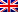# Mass, Scalar Curvature, Kähler Geometry, and All That

Given a complete Riemannian manifold that looks enough like Euclidean space at infinity, physicists have defined a quantity called the “mass” that measures the asymptotic deviation of the geometry from the Euclidean model. After first providing a self-contained introduction to the key underlying geometric concepts, I will go on to explain a simple formula, discovered in joint work with Hajo Hein, for the mass of any asymptotically locally Euclidean (ALE) Kähler manifold. When the metric is actually AE (asymptotically Euclidean), our formula not only implies the positive mass theorem for Kähler metrics, but also yields a Penrose-type inequality for the mass. I will also briefly indicate some recent technical improvements that allow one to prove these results assuming only minimal metric fall-off assumptions at infinity.
This post is also available in: Link identifier #identifier__49005-5Eng